Next: Conservation of energy Up: Newton's laws of motion Previous: Worked example 4.3: Raising

## Worked example 4.4: Suspended block

Question: Consider the diagram. The mass of blockis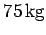and the mass of blockis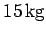. The coefficient of static friction between the two blocks is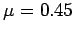. The horizontal surface is frictionless. What minimum forcemust be exerted on blockin order to prevent blockfrom falling?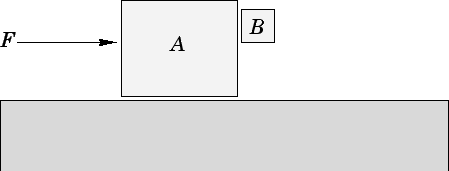Answer: Suppose that blockexerts a rightward forceon block. By Newton's third law, blockexerts an equal and opposite force on block. Applying Newton's second law of motion to the rightward accelerationof block, we obtain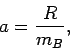where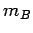is the mass of block. The normal reaction at the interface between the two blocks is. Hence, the maximum frictional force that blockcan exert on blockis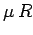. In order to prevent blockfrom falling, this maximum frictional force (which acts upwards) must exceed the downward acting weight,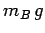, of the block. Hence, we require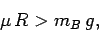or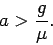Applying Newton's second law to the rightward accelerationof both blocks (remembering that the equal and opposite forces exerted between the blocks cancel one another out), we obtain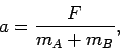where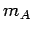is the mass of block. It follows that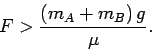Since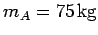,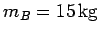, and, we have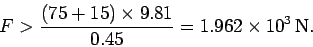Next: Conservation of energy Up: Newton's laws of motion Previous: Worked example 4.3: Raising
Richard Fitzpatrick 2006-02-02